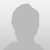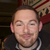+1

# eval statement for comparing times

jenifercmartin 7 years ago updated by icahill (Administrator) 7 years ago
I want app users to be able to input their best time for an event and then have the app compare that time to a set standard time so that the user can see how far over or under he is.

the data is in format m:ss.00, which I formatted (as a custom format) in the excel sheet i used to build the database. When I built the SQLite database, I put a "text" data type on the columns with the times in them. How would i compute the difference between, say, 2:27.6 and 2:22.00 ? or 1:00.5 and 0:57.99?Jenifer,

John created this js function that you can use to achieve this.

function subtractTime(time,time2)
{
time = time.replace("\.",":");
time2 = time2.replace("\.",":");
var t1 = time.split(":");
var t2 = time2.split(":");
var t1ms = parseInt(t1 * 60000) + parseInt(t1 * 1000)
+ parseInt(t1);
var t2ms = parseInt(t2 * 60000) + parseInt(t2 * 1000)
+ parseInt(t2);
var tfinal = Math.abs(t1ms - t2ms);
var ms = tfinal % 1000;
tfinal = (tfinal - ms) / 1000;
var secs = tfinal % 60;
tfinal = (tfinal - secs) / 60;
var mins = tfinal % 60;
var hrs = (tfinal - mins) / 60;
return (mins<10? '0':'') + mins + ':' + (secs<10? '0':'') +
secs + '.' + ms;
}

To use this, your eval will look like this: [eval: subtractTime('0:58.02','1:57.00');]Thanks, Ian and John! You made my day.Ian and John,
Creating a homework app and I want to do an eval to calculate current date - due date and lets say if its less than 1 day, it changes background to red, between 2 and 3 days it changes color to yellow and greater than or equal to 4 its green. Is there a JS calculator to subtract dates, using the default format of yyyy-mm-dd.``` <wire> <main> <pager name="pager" width="100%" height="100%" orientation="vertical" paginate="no"> <text name="Test-1" width="100%" height="100" color="#ffffff" font="Arial" size="48" align="center" alignment="center" text="getDate is [eval: new Date().getDate(); ] "></text> <text name="Test-2" width="100%" height="100" color="#ffffff" font="Arial" size="48" align="center" alignment="center" text="getMonth +1 is [eval: 1+ new Date().getMonth(); ] "></text> <text name="Test-3" width="100%" height="100" color="#ffffff" font="Arial" size="48" align="center" alignment="center" text="getDay is [eval: new Date().getDay(); ] "></text> <text name="Test-4" width="100%" height="100" color="#ffffff" font="Arial" size="48" align="center" alignment="center" text="HEY is[eval: new Date().getMonth();]-[eval: new Date().getDate();]-[eval: new Date().getFullYear();] "></text> <text name="Test-5" width="100%" height="100" color="#ffffff" font="Arial" size="48" align="center" alignment="center" text="HEY is [eval: [eval: new Date().getDate();] - 4]"></text> </pager> </main> <action name="on" oninit="yes"> <assign property="var:today" value="[eval: new Date().getMonth();]-[eval: new Date().getDate();]-[eval: new Date().getFullYear();]" /> <assign property="var:difference" value="[eval: [eval: new Date().getDate();] - 4]" /> <assign property="var:new" value="[eval: new Date().getMonth();]-[var:difference]-[eval: new Date().getFullYear();]" /> <alert message="[var:new]"/> </action> </wire> ```